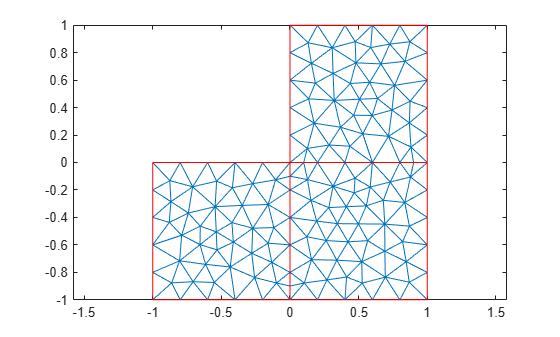# initmesh

Create initial 2-D mesh

This page describes the legacy workflow. New features might not be compatible with the legacy workflow. For the corresponding step in the recommended workflow, see `generateMesh`.

## Syntax

``[p,e,t] = initmesh(g)``
``[p,e,t] = initmesh(g,Name,Value)``

## Description

example

````[p,e,t] = initmesh(g)` generates a triangular mesh for a 2-D geometry. The function uses a Delaunay triangulation algorithm.```

example

````[p,e,t] = initmesh(g,Name,Value)` generates a 2-D mesh using one or more `Name,Value` pair arguments.```

## Examples

collapse all

Generate a triangular mesh of the L-shaped membrane.

`[p,e,t] = initmesh('lshapeg');`

Plot the mesh.

`pdemesh(p,e,t)`Generate a triangular mesh of the L-shaped membrane with the target maximum mesh edge length of 0.1.

`[p,e,t] = initmesh('lshapeg','Hmax',0.1);`

Plot the mesh.

`pdemesh(p,e,t)`## Input Arguments

collapse all

Geometry description, specified as a decomposed geometry matrix, a geometry function, or a handle to the geometry function. For details about a decomposed geometry matrix, see `decsg`. For details about a geometry function, see Parametrized Function for 2-D Geometry Creation.

A geometry function must return the same result for the same input arguments in every function call. Thus, it must not contain functions and expressions designed to return a variety of results, such as random number generators.

Data Types: `double` | `char` | `string` | `function_handle`

### Name-Value Arguments

Specify optional comma-separated pairs of `Name,Value` arguments. `Name` is the argument name and `Value` is the corresponding value. `Name` must appear inside quotes. You can specify several name and value pair arguments in any order as `Name1,Value1,...,NameN,ValueN`.

Example: `[p,e,t] = initmesh('lshapeg','Hmax',0.1);`

Target maximum mesh edge length, specified as the comma-separated pair consisting of `'Hmax'` and a positive real number.

`Hmax` is an approximate upper bound on the mesh edge lengths. `initmesh` estimates the default value of `Hmax` from overall dimensions of the geometry.

Small `Hmax` values let you create finer meshes, but mesh generation can take a very long time in this case. You can interrupt mesh generation by using Ctrl+C. Note that `initmesh` can take additional time to respond to the interrupt.

Example: `[p,e,t] = initmesh(g,'Hmax',0.25);`

Data Types: `double`

Mesh growth rate, specified as the comma-separated pair consisting of `'Hgrad'` and a number strictly greater than 1 and less than 2.

Example: `[p,e,t] = initmesh(g,'Hgrad',1.5);`

Data Types: `double`

Toggle to preserve bounding box, specified as the comma-separated pair consisting of `'Box'` and `'on'` or `'off'`. By turning on `'Box'` you can get a good idea of how the mesh generation algorithm works within the bounding box.

Example: `[p,e,t] = initmesh(g,'Box','on');`

Data Types: `char` | `string`

Toggle to use edge triangulation, specified as the comma-separated pair consisting of `'Init'` and `'on'` or `'off'`. By turning on `Init` you can see the initial triangulation of the boundaries. For example, use these commands to determine the subdomain number `n` of the point `xy`.

```[p,e,t] = initmesh(g,'Hmax',Inf,'Init','on'); [uxy,tn,a2,a3] = tri2grid(p,t,zeros(size(p,2)),x,y); n = t(4,tn); ```

If the point is outside the geometry, `tn` is `NaN`, and the command `n = t(4,tn)` results in a failure.

Data Types: `char` | `string`

Toggle to call `jigglemesh` after creating the mesh, specified as the comma-separated pair consisting of `'Jiggle'` and `'mean'`, `'minimum'``'on'`, or `'off'`.

• `'mean'` — call `jigglemesh` with the argument `'Opt'` set to `'mean'`.

• `'minimum'` — call `jigglemesh` with the argument `'Opt'` set to `'minimum'`.

• `'on'` — call `jigglemesh` with the argument `'Opt'` set to `'off'`.

• `'off'` — do not call `jigglemesh`.

Example: ```[p,e,t] = initmesh(g,'Jiggle','minimum');```

Data Types: `char` | `string`

Maximum number of iterations for `jigglemesh`, specified as the comma-separated pair consisting of `'JiggleIter'` and a positive integer.

Example: ```[p,e,t] = initmesh(g,'Jiggle','on','JiggleIter',50);```

Data Types: `double`

Algorithm for generating initial mesh, specified as the comma-separated pair consisting of `'MesherVersion'` and either `'R2013a'` or `'preR2013a'`. The `'R2013a'` algorithm runs faster, and can triangulate more geometries than the `'preR2013a'` algorithm. Both algorithms use Delaunay triangulation.

Data Types: `char` | `string`

## Output Arguments

collapse all

Mesh points, returned as a 2-by-`Np` matrix. `Np` is the number of points (nodes) in the mesh. Column `k` of `p` consists of the x-coordinate of point `k` in `p(1,k)` and the y-coordinate of point `k` in `p(2,k)`. For details, see Mesh Data as [p,e,t] Triples.

Mesh edges, returned as a 7-by-`Ne` matrix, where `Ne` is the number of boundary edges in the mesh. An edge is a pair of points in `p` containing a boundary between subdomains, or containing an outer boundary. For details, see Mesh Data as [p,e,t] Triples.

Mesh elements, returned as a 4-by-`Nt` matrix. `Nt` is the number of triangles in the mesh.

The `t(i,k)`, with `i` ranging from 1 through `end - 1`, contain indices to the corner points of element `k`. For details, see Mesh Data as [p,e,t] Triples. The last row, `t(end,k)`, contains the subdomain number of the element.

 George, P. L. Automatic Mesh Generation — Application to Finite Element Methods. Wiley, 1991.

## Support#### Try MATLAB, Simulink, and Other Products

Get trial now